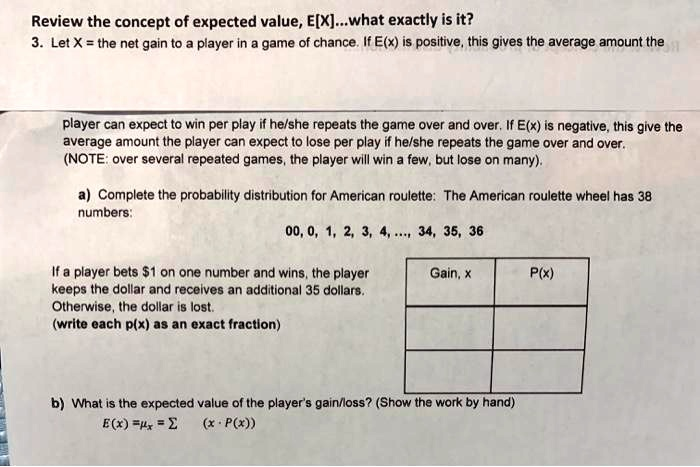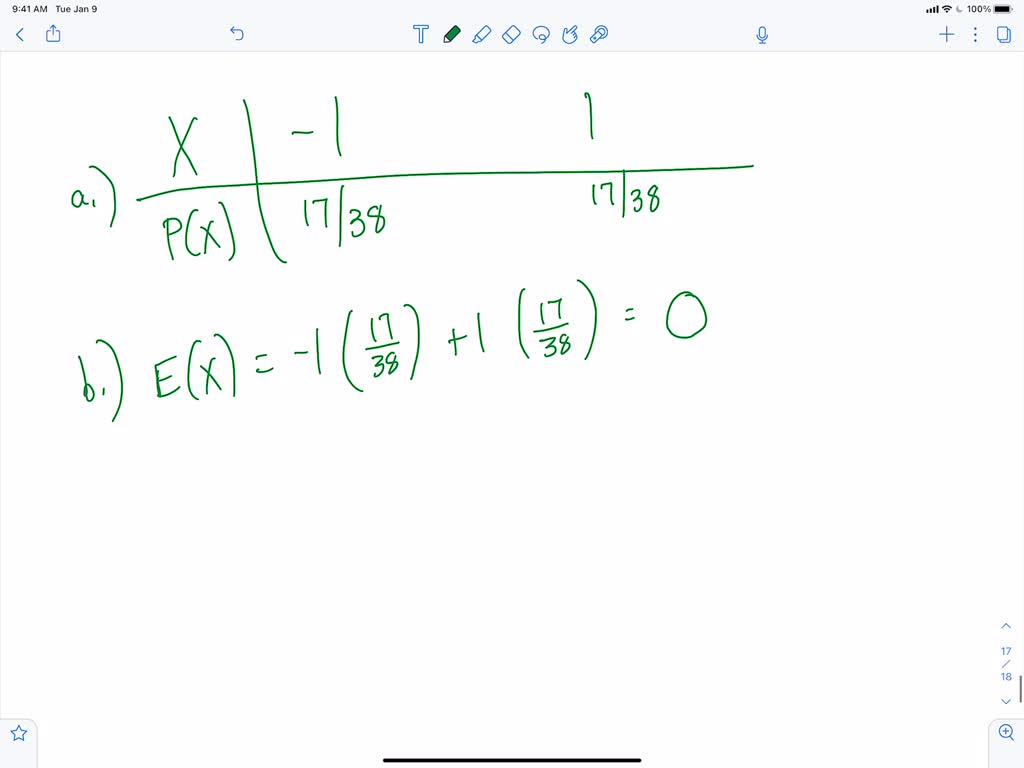5

# Review the concept of expected value; E[X]_what exactly is it? Let X = the net gain to player in game of chance: If E(x) is positive, this gives the average amount ...

## Question

###### Review the concept of expected value; E[X]_what exactly is it? Let X = the net gain to player in game of chance: If E(x) is positive, this gives the average amount theplayer can expect to win per play if helshe repeats the game over and over, If E(x) is negative, this give the average amount the player can expect t0 lose per play if helshe repeats the game over and over. (NOTE over several repeated games the player wlll win few; but lose on many):a) Complete the probability distribution for Ame

Review the concept of expected value; E[X]_what exactly is it? Let X = the net gain to player in game of chance: If E(x) is positive, this gives the average amount the player can expect to win per play if helshe repeats the game over and over, If E(x) is negative, this give the average amount the player can expect t0 lose per play if helshe repeats the game over and over. (NOTE over several repeated games the player wlll win few; but lose on many): a) Complete the probability distribution for American roulette: The American roulette wheel has 38 numbers" 00, 0, 1, 2 34, 35, 36 If a player bets $1 on one number and wins_ the player keeps the dollar and recelves an additional 35 dollars Otherwise , Ihe dollar I8 lost. (write each P(x) asan exact fractlon) Gain; P(x) What is the expected value of the player$ gainiloss? (Show the work by hand) E(x) =lr (x " P())